# Simona Riva

•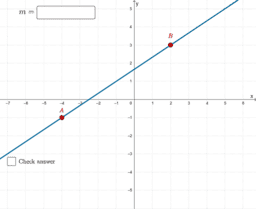### Linear Functions and Rate of Change - work in progress

Activity

Simona Riva

•### Coordinate Geometry

Book

Simona Riva

•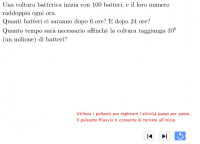### A Simple Exponential Model in the Time of Covid

Activity

Simona Riva

•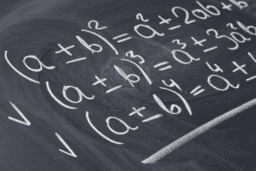### Pre-Algebra and Algebra

Book

Simona Riva

•### Modeling with Functions

Book

Simona Riva

•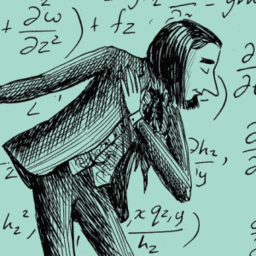### Calculus

Book

Simona Riva

•### dms → decimal angle converter

Activity

Simona Riva

•### Complex numbers: rectangular and polar form

Activity

Simona Riva

•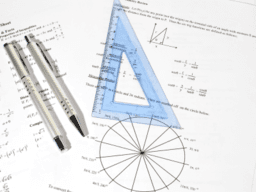### Trigonometry and Complex Numbers

Book

Simona Riva

•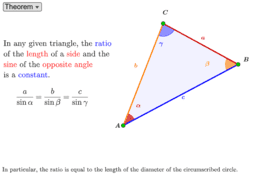### Lesson and Practice: The Law of Sines

Activity

Simona Riva

•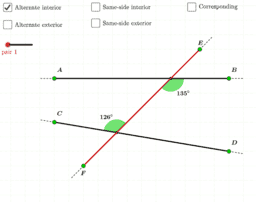### Lesson and Practice: Angles Between Two Lines and a Transversal

Activity

Simona Riva

•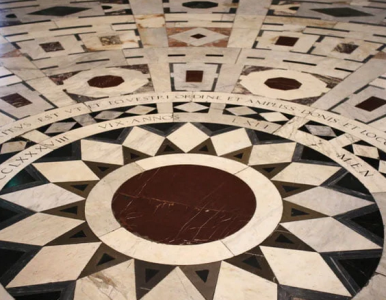### Euclidean Geometry

Book

Simona Riva

•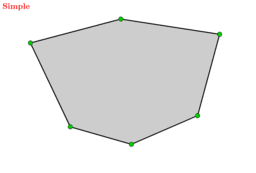### Lesson and Practice: Convex, Concave and Self-Intersecting Polygons

Activity

Simona Riva

•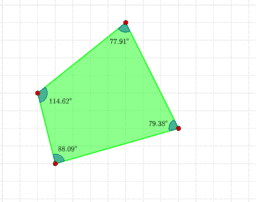### The (mathematical) logic behind the scenes

Activity

Simona Riva

•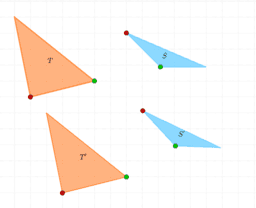### Counterexamples

Activity

Simona Riva

•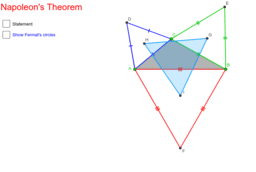### Explore! Napoleon's Theorem - Defining Hypothesis and Thesis

Activity

Simona Riva

•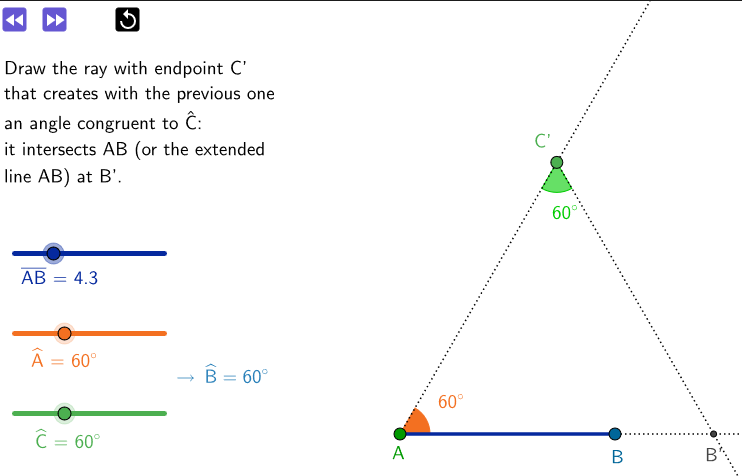### Lesson: Construction of a triangle, given a side and two angles

Activity

Simona Riva

•### Explore! Il puzzle di Haberdasher - Haberdasher's puzzle

Activity

Simona Riva

•### Lesson and Practice: Special Points of Triangles

Activity

Simona Riva

•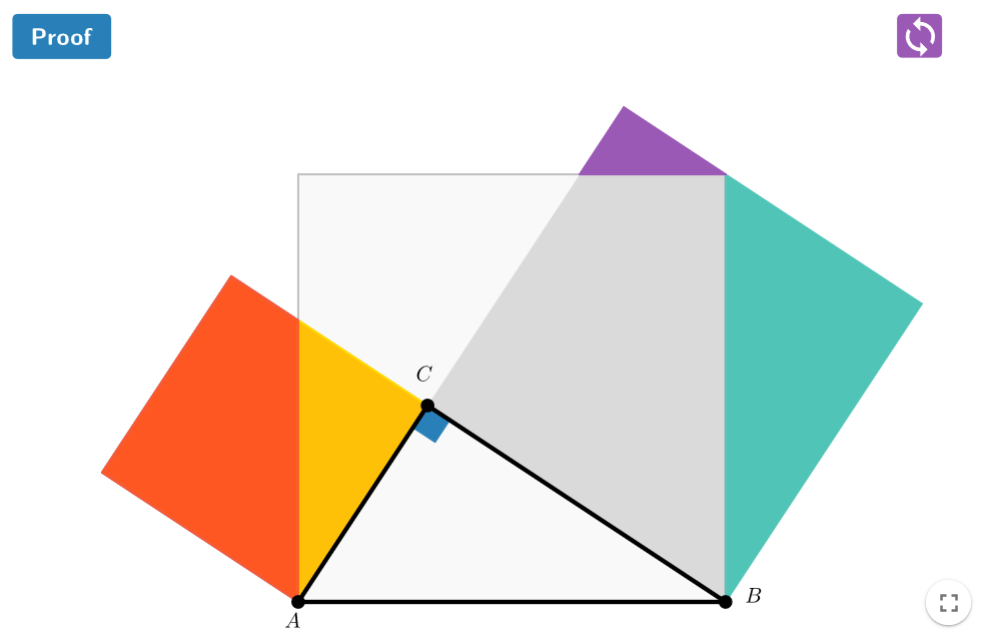### Lesson: Pythagorean Theorem - a "proof without words"

Activity

Simona Riva

•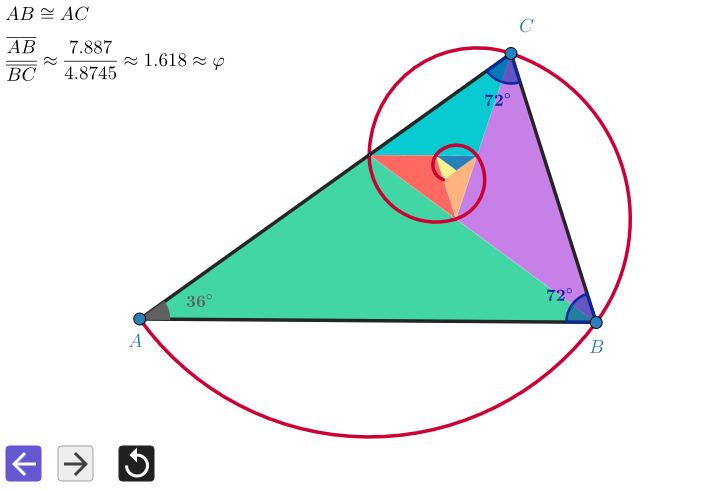### Lesson: Golden Triangle and Spiral

Activity

Simona Riva

•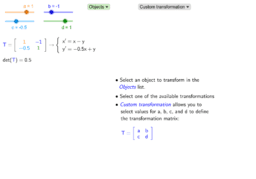### Lesson & Practice: Matrices and Linear Transformations

Activity

Simona Riva

•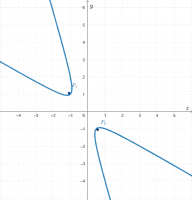### Lesson: Conics and Euclidean Invariants

Activity

Simona Riva

•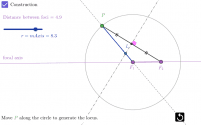Activity

Simona Riva